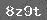# 笔算乘法习题精选

1．直接写得数

28×3=　　 270×3=　　 8×6＋5=

49×2＝　　160×4＝　　7×8＋4＝

17×40=　　190×5=　　 9×4＋5=

23×20＝　 14×50＝　　5×9＋7=

2．计算下列各题18×34＝　　　19×25＝　　27×32＝　　45×12＝

3．列式计算

（1）13个34是多少？

（2）26的32信是多少？

（3）把43连续加19次，结果是多少？

（4）23和16相乘，积是多少？

1. 计算题2. 计算题

33×12=     232×6=      23×31=

3. 计算题

142×23=     32×37=

4. 甲数比乙数的2倍多126，乙数是25，求甲数.

5. 23个35是多少?

1. 填空

用乘数个位上的数与被乘数相乘,得到的积是表示几个一;用乘数十位上的数与被乘数相乘,得到的积是表示几个十. ( )

2. 计算题

114×25=　　433×23=　　25×43=　　302×24=

3. 比45的13倍少205的数是多少?

4. 14的25倍是多少?

### 相关推荐

 留言与评论（共有 0 条评论）

 验证码：